## Tangent Plane – Step by Step – using the TiNspire CX

QUESTION:  Find an equation of the tangent plane to the surface
z=3x^4+9y^4+7xy at the point (3,3,1035).

SOLUTION: Start Calculus Made Easy , go to the Multivariable Calculus in the menu.

There, enter as shown below :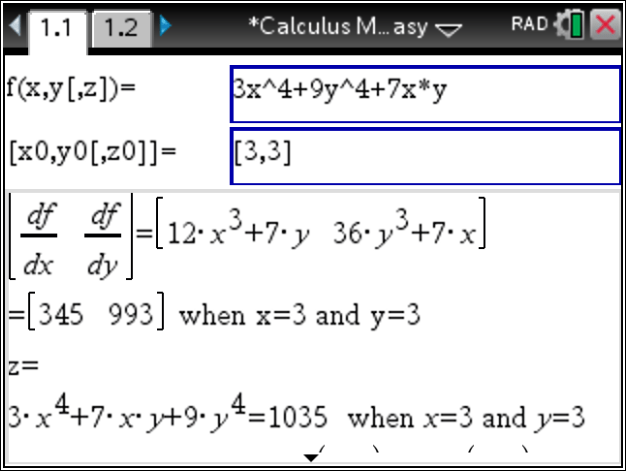The steps are shown in the box below: partial derivatives are computed and evaluated. And the function is evaluated at the given point . Thus, the Tangent Plane is derived. Additionally, a line normal to the plane and a normal vector are found.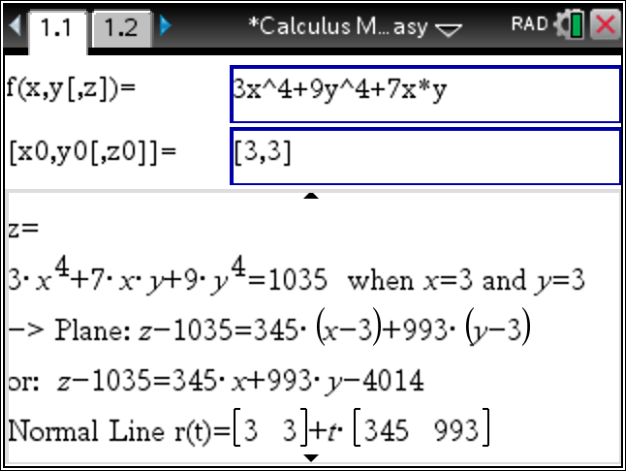Thus, finally
Posted on Categories calculus

## Ti NSpire CX: Implicit Differentiation – Step by Step

Using Calculus Made Easy on the TiNSpire CX at  https://www.tinspireapps.com/?a=CME

choose IMPLICIT DIFFERENTIATION in the menu , then enter  the equation of a

given curve as shown below:The Implicit Differentiation process continues until step 5)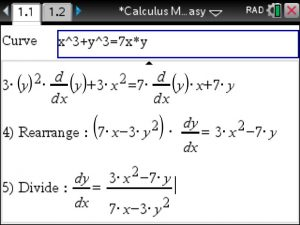VOILA ! easy as pie! The handy TiNspire CX can not only Implicit Differentiation Step by Step but can do ALL

of Calculus Step by Step!

Posted on Categories calculus, tinspirecx

## Confidence Intervals and Margin of Error using the TiNSpire CX

To find Confidence Intervals of Proportions using Statistics Made Easy at  https://www.tinspireapps.com/?a=SPME use option 7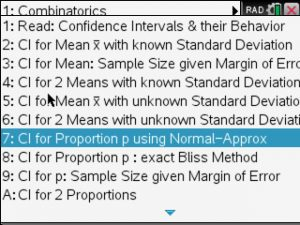Next, the given proportion po , sample size n and the confidence level alpha as shown below: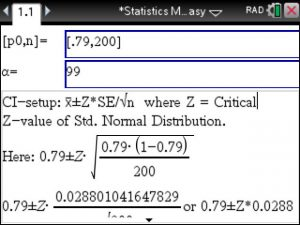Automatically, the correct setup and solution shows in the bottom window.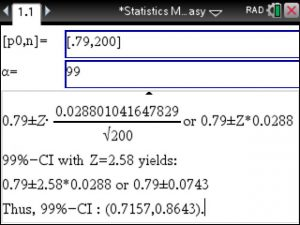To also find the sample size given the Margin of Error use option 9: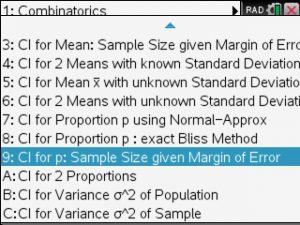you will enter the given Margin of Error, proportion and the confidence level Alpha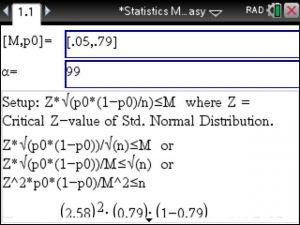Again, the correct setup is shown and the sample size is computed step by step, here we get n>=441.7187Posted on Categories statistics

## ▷[Solved] Compute Limits in Calculus – Step by Step – using the TiNspire CX CAS

In your Calculus class you will be asked to compute limits. You should know that there is a variety of techniques to figure out limits such as via Factoring, via Common Denominator and of course via L’Hopital rule (which we covered in earlier posts already) . All these techniques can be applied using the Calculus Made Easy app , just navigate to Limits in the Menu as seen below:When selecting the menu option 4: Limit via Factoring you enter your function and the x value that you are approaching. Now lean back and observe the steps to arrive at the limit. Calculus all of a sudden got easy! See below.Another common method to compute Limits is via Common Denominator of the numerator. Read the sentence again to digest it better 😉

The tricky part is to enter the two fractions in the numerator properly, extra set of parentheses are needed here. See belowThe steps to compute the limits are displayed below: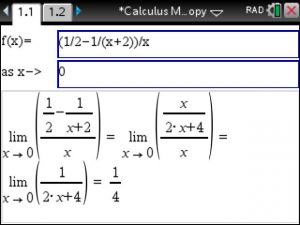These Step by Step Limit computation methods using your TiNspire CX CAS will allow you to master your Calculus class.

Posted on Categories calculus, Limits, solver, tinspirecx

## ▷Riemann Sums – Step by Step – with the TiNSpire CX CAS: Calculus Made Easy

Say you need to compute Riemann Sums at selected points over a given interval. And you do have your trusty TiNspire CX on you. Then start Calculus Made Easy , go to option 1 J in the menu and enter

1. the given function
2. the given interval
3. the selected x-values c1, c2, ..

as shown in image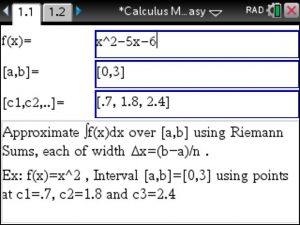Then each Riemann rectangle is computed step by step as shown below :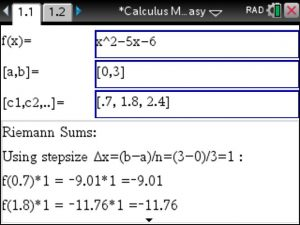Lastly, view the final Riemann Sum and compare it to the exact definite integral. Pretty close 😉Posted on Categories calculus, Integral

## ▷Relative Rate of Change using the TiNspire CX CAS

To find the Relative Rate of Change using the TiNSpire CX CAS open up Calculus Made Easy from www.TiNspireCX.com , then go menu item “Derivatives” and select “Relative Rate of Change”. Enter the given Function and Voila, the Relative Rate of Change shows immediately, Very simple to use !!!

Remember that Relative Rate of Change in Calculus is the Derivative of a Function divided by the Function itself. Here is an example:

f(x) = 4x + exp(7x) , then f'(x) = 4 + 7*exp(7x) ,

Thus , Relative Rate of Change of f =

(4 + 7*exp(7x) ) / (4x + exp(7x) )

Posted on Categories calculus

## ▷Everything works like a charm. Just purchased the statistics app for the TiNSpire CX

Hey Mike!

All is well!

You helped me over text a week ago!

Everything works like a charm.

Just purchased the statistics app and just activated

That works as well.

Posted on Categories customer feedback

## Mean, Mode, Median, Standard Deviation of LARGE data sets using the TiNspire CX

Say you have 10 thirteens, 10 twos and 500 threes and need to find

Mean, Mode, Median, Standard Deviation, Variance etc. To avoid looooots of typing you can

now enter such LARGE data using the TiNspire’s Statistics Made Easy app at www.TiNspireApps.com

Enter data as simple as : [10,10,500]   and [13,2,3]

Voila! That simple!

We love your suggestions, keep them coming. This feature was suggested by user Steve L. GREAT suggestion!

Posted on Categories statistics